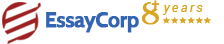WhatsApp
Struggling With Assignments?

We are here to help.

Chat with our expert writers on WhatsApp

for any kind of assignment queries.

Click to chat!
Struggling with Assignments? WhatsApp Us#### Question :

The first part should be solved manually, and the second part should be done by using abaqus software. Consider a first-order triangular element, if we know the coordinates (x,y) and the displacements (u,v) at the nodes as follows: Node 1: (x1, y1) = (3.0,3.0), (u1, v1) = (0.012,0.006) m Node 2: (x2, y2) = (2.0,4.0), (u2, v2) = (0.015,0.005) m Node 3: (x3, y3) = (1.0,1.0), (u3, v3) = (0.010,0.008) m a) Find the interpolation functions for this element. b) Calculate the displacements (u, v) at the points (4, 3) and (3, 2).

#### Solution :HIRE EXPERT
Email ID *
Subject
Pages